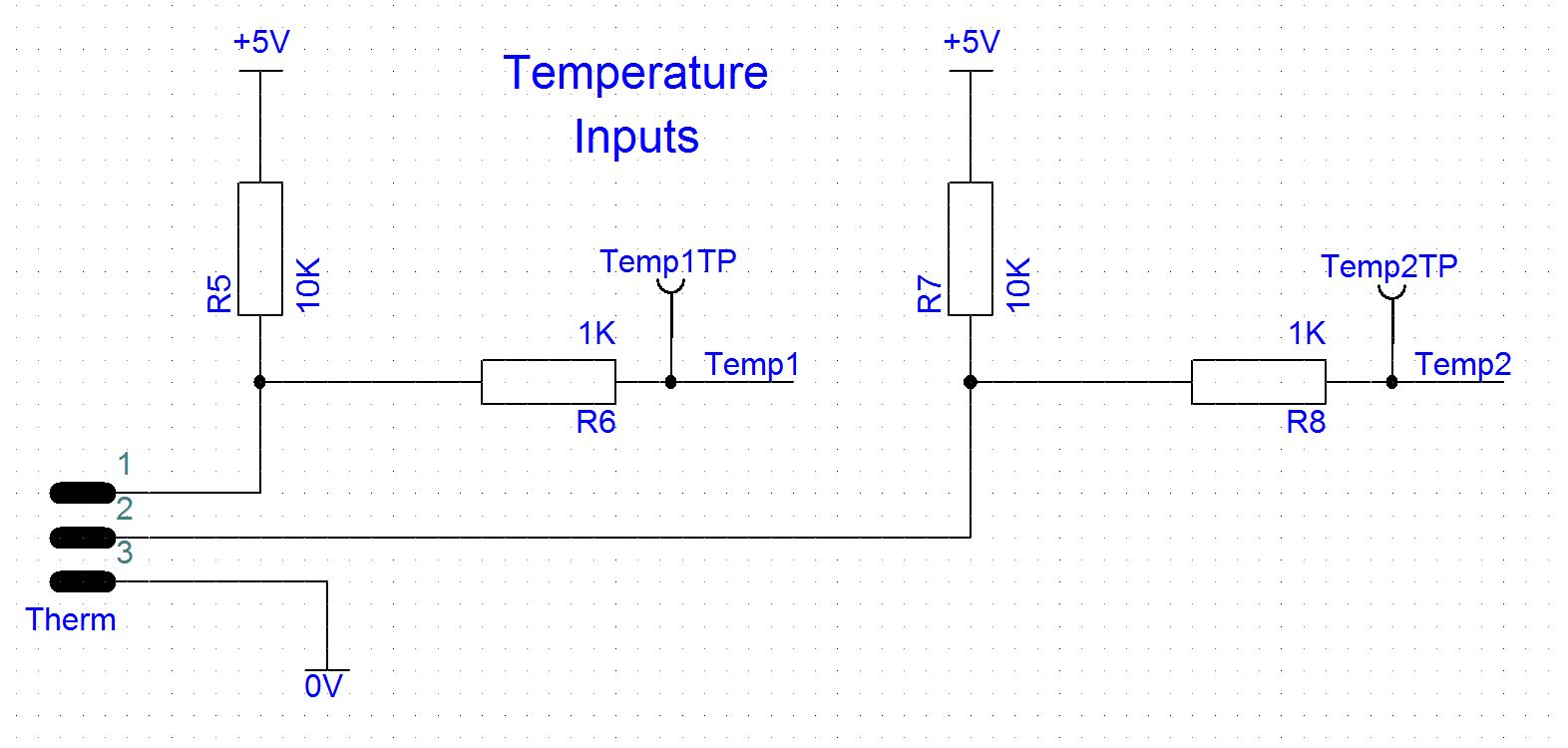# Temperature Inputs

The thermistors form part of a potential divider. The resistance of the thermistor changes with its temperature. As the resistance changes the output voltage of the potential divider changes.In the diagram above, the ‘Therm’ component to the left represents the three pin connector on the eChook board. Thermistor one is connected between pins 1 and 3, and thermistor two is connected between pins 2 and 3, completing potential dividers with R5 and R7 respectively.
R6 and R8 are in place to protect the Arduino. The Arduino can be easily damaged by too much voltage and without these resistors the pins would be directly connected to an external connector, making them vulnerable. The 1k resistor provides a little protection, although 24v applied to the connector will still damage the Arduino. They also do not affect the reading from the temperature sensor, as the current flowing into the Arduinos Analog to Digital Converter (ADC) is so negligeable that it can be assumed to be 0mA. The voltage drop across the resistor can be calculated by Ohm’s law, V=I*R. In this case, V = 0 * 1000, therefore V = 0.